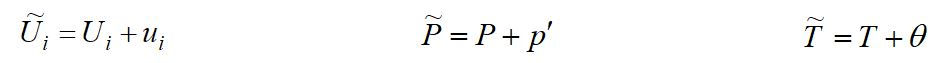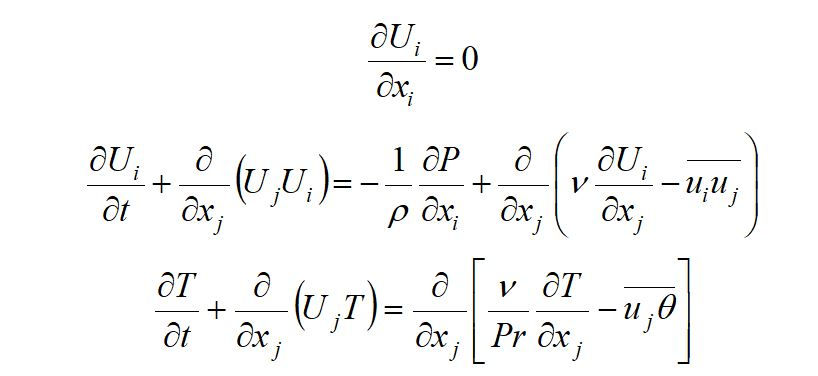top of page
Search
• ManchesterCFD Amin

# What’s all the fuss about ‘Turbulence Models’ in CFD

1. Turbulence Model

A turbulence model is a mathematical formulation used to close the system of mean flow equations. It is desirable that a turbulence model should have a broad range of applicability, while also being as simple as possible.

For most engineering purposes it is unnecessary to resolve the details of the turbulent fluctuations and only a statistical description of the flow, and the effects of the turbulence on the mean flow are usually sought.

As was discussed in the previous lectures, one of the most common methods of computationally modelling turbulent flows is known as the ‘Reynolds Averaged Navier-Stokes’ (RANS) approach which is based on time-averaging the Navier-Stokes equations.

The Reynolds decomposition of the instantaneous velocities, pressure and temperature into averaged and fluctuating parts can be written aswhere the upper case symbols represent the mean quantities and corresponds to the fluctuating part.

As we saw earlier, by taking the average of Navier-Stokes equations and replacing the flow variables in these equations by the mean and fluctuating parts (obtained by the Reynolds decomposition), the RANS equations are obtained asFor example, for two-dimensional, incompressible and steady flows, the above equations are re-written as follows:The left-hand sides of Eqns. (79) and (80) represent the convective terms, while the right-hand sides consist of the pressure gradient, the diffusive terms (containing viscous effects), and the turbulent Reynolds stresses.

One of the drawbacks of RANS equations is that information is lost in the averaging process which gives rise to the appearance of the Reynolds stress tensor and thus, it is the role of turbulence models to provide an approximation to these terms.

Similarly, the turbulent heat fluxes which appear in the Energy Equation, Eqn. (81) need to be modeled too.

Generally, there are two major types of turbulence models for closing RANS equations: Eddy Viscosity Models (EVMs) and Reynolds Stress Models (RSMs). The basis for the vast majority of common turbulence models stems from the Eddy Viscosity model which is discussed below. In an Eddy Viscosity Model, the Reynolds stresses and turbulent heat fluxes are directly linked to the local gradients of the mean flow field through a turbulent viscosity and diffusivity, where the turbulent viscosity itself is determined by a characteristic velocity- and length-scale. The choice of scales is wide, ranging from prescribed diffusivity profiles to widely-used two-equation models.

## Recent Posts

See All

1. Modes of Heat Transfer Conduction, convection and radiation are the three mechanisms by which thermal energy may be transferred from one point in space (and time) to another. • Conduction: diffusio

bottom of page